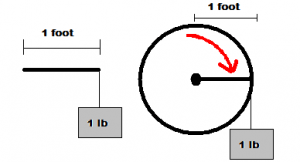# Torque. It’s Not a New Twist on Power

Torque can be a difficult force to describe.  If I had to use another word to describe it, I would use the word twist.  Without understanding what torque is, and how it is used, we can’t really understand many other mechanical functions, like horsepower.

When I think of an example of torque, I think about arm wrestling.  It’s hard to imagine someone not knowing what arm wrestling is, so I’ll assume you know.  When you look at one of the opponents, he is trying to twist the other person’s arm down.  This twisting motion is torque.  If you think about it, he is applying force at his elbow, and then his arm is a lever.  Those are the two factors that describe it, a force for a given length of lever arm.

Maybe I got ahead of myself.  Let’s look at how torque is described in science.  In the US we use units of measurement called inch-pounds, or foot-pounds.  Sometimes these are described as in/lbs, or ft/lbs.  Basically the unit is self-descriptive.  For foot-pounds, imagine a 1 foot wrench on a bolt.  If you apply one pound of pressure at the opposite end of that wrench, you are applying 1 ft/lbs of torque on that bolt.  If the imaginary wrench was 1 inch long, you would be applying 1 in/lbs of torque.

I like using the imaginary wrench comparison to try to explain torque.  It also works with a wheel that has a radius of 1 foot.  If you put one pound of force tangent to the circumference of the wheel, you would be applying one ft/lb of torque.If you have a 3 foot imaginary wrench and you apply 10 pounds of force to the end of that wrench, how many ft/lbs of torque are you applying?  3 x 10 = 30 ft/lbs.

Torque is one of the variables used to calculate mechanical horsepower.  The other is RPM.

What other places do you see torque applied?  Remember to look for twisting motion.  Can you estimate how much torque is being applied?

For more calculations of torque, try these online calculators:

Inch Pound Torque Conversion Calculator

Mechanical Horsepower Calculator

Gear Increaser Calculator

Gear Reducer Calculator

CalcuNATION is a website featuring online calculators and educational resources for mathematics.  Other Mathematical Blogs ( CalcuNATION on EduBlogs and CalcuNATION on Blogger)

Torque. It’s Not a New Twist on Power
Scroll to top